Successfully reported this slideshow.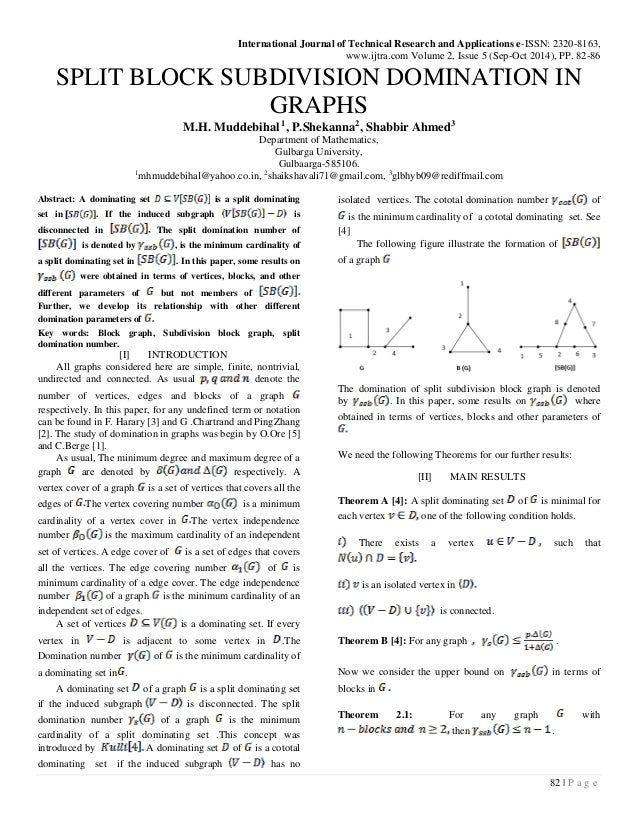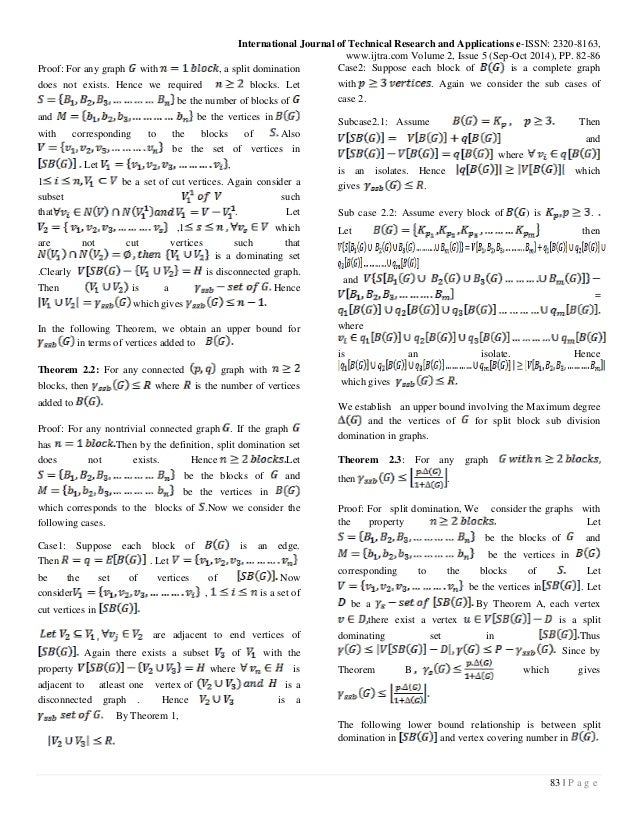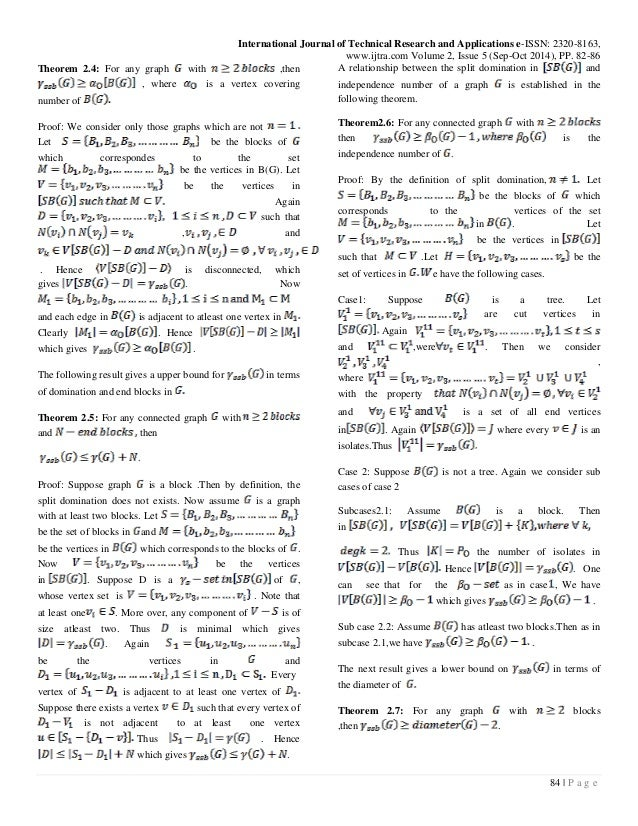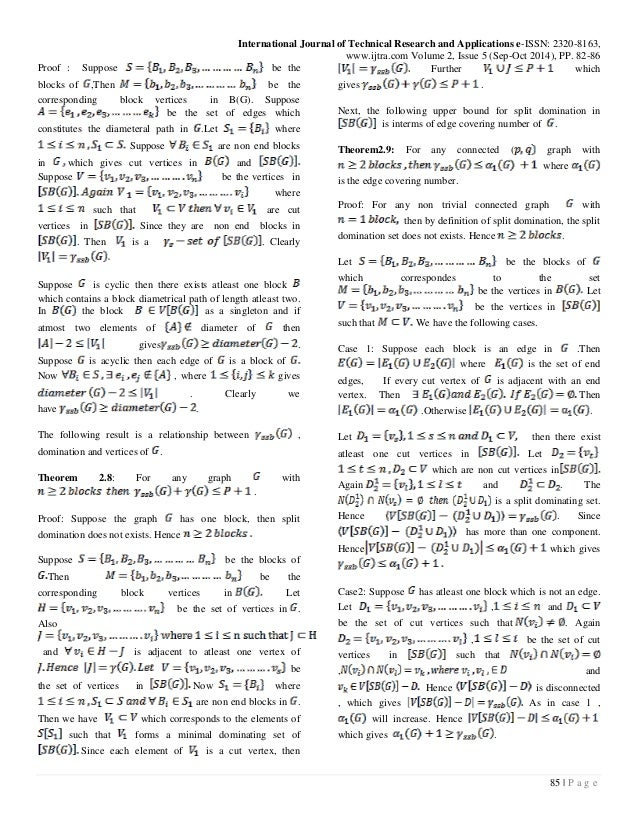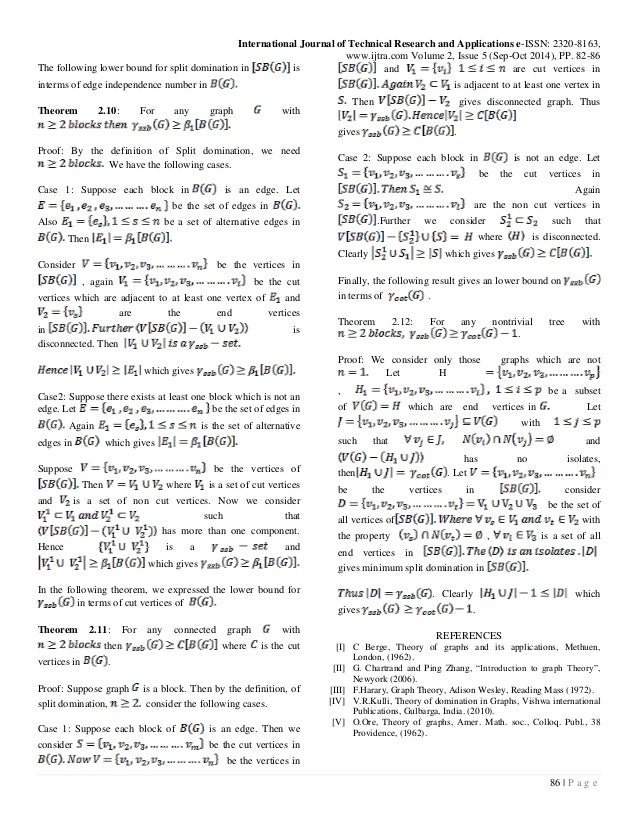Upcoming SlideShare
×

0

Share

# SPLIT BLOCK SUBDIVISION DOMINATION IN GRAPHS

A dominating set is a split dominating
set in . If the induced subgraph is
disconnected in The split domination number of
is denoted by , is the minimum cardinality of
a split dominating set in . In this paper, some results on
were obtained in terms of vertices, blocks, and other
different parameters of but not members of
Further, we develop its relationship with other different
domination parameters of

• Full Name
Comment goes here.

Are you sure you want to Yes No• Be the first to comment

• Be the first to like this

### SPLIT BLOCK SUBDIVISION DOMINATION IN GRAPHS

1. 1. International Journal of Technical Research and Applications e-ISSN: 2320-8163, www.ijtra.com Volume 2, Issue 5 (Sep-Oct 2014), PP. 82-86 82 | P a g e SPLIT BLOCK SUBDIVISION DOMINATION IN GRAPHS M.H. Muddebihal 1, P.Shekanna2, Shabbir Ahmed3 Department of Mathematics, Gulbarga University, Gulbaarga-585106. 1 mhmuddebihal@yahoo.co.in, 2 shaikshavali71@gmail.com, 3 glbhyb09@rediffmail.com Abstract: A dominating set is a split dominating set in . If the induced subgraph is disconnected in The split domination number of is denoted by , is the minimum cardinality of a split dominating set in . In this paper, some results on were obtained in terms of vertices, blocks, and other different parameters of but not members of Further, we develop its relationship with other different domination parameters of Key words: Block graph, Subdivision block graph, split domination number. [I] INTRODUCTION All graphs considered here are simple, finite, nontrivial, undirected and connected. As usual denote the number of vertices, edges and blocks of a graph respectively. In this paper, for any undefined term or notation can be found in F. Harary  and G .Chartrand and PingZhang . The study of domination in graphs was begin by O.Ore  and C.Berge . As usual, The minimum degree and maximum degree of a graph are denoted by respectively. A vertex cover of a graph is a set of vertices that covers all the edges of The vertex covering number is a minimum cardinality of a vertex cover in The vertex independence number is the maximum cardinality of an independent set of vertices. A edge cover of is a set of edges that covers all the vertices. The edge covering number of is minimum cardinality of a edge cover. The edge independence number of a graph is the minimum cardinality of an independent set of edges. A set of vertices is a dominating set. If every vertex in is adjacent to some vertex in .The Domination number of is the minimum cardinality of a dominating set in . A dominating set of a graph is a split dominating set if the induced subgraph is disconnected. The split domination number of a graph is the minimum cardinality of a split dominating set .This concept was introduced by A dominating set of is a cototal dominating set if the induced subgraph has no isolated vertices. The cototal domination number of is the minimum cardinality of a cototal dominating set. See  The following figure illustrate the formation of of a graph The domination of split subdivision block graph is denoted by . In this paper, some results on where obtained in terms of vertices, blocks and other parameters of We need the following Theorems for our further results: [II] MAIN RESULTS Theorem A : A split dominating set of is minimal for each vertex one of the following condition holds. There exists a vertex such that is an isolated vertex in is connected. Theorem B : For any graph . Now we consider the upper bound on in terms of blocks in Theorem 2.1: For any graph with then .
2. 2. International Journal of Technical Research and Applications e-ISSN: 2320-8163, www.ijtra.com Volume 2, Issue 5 (Sep-Oct 2014), PP. 82-86 83 | P a g e Proof: For any graph with , a split domination does not exists. Hence we required blocks. Let be the number of blocks of and be the vertices in with corresponding to the blocks of Also be the set of vertices in Let , 1 be a set of cut vertices. Again consider a subset such that . Let ,1 which are not cut vertices such that is a dominating set .Clearly is disconnected graph. Then is a Hence which gives In the following Theorem, we obtain an upper bound for in terms of vertices added to Theorem 2.2: For any connected graph with blocks, then where is the number of vertices added to Proof: For any nontrivial connected graph . If the graph has Then by the definition, split domination set does not exists. Hence Let be the blocks of and be the vertices in which corresponds to the blocks of .Now we consider the following cases. Case1: Suppose each block of is an edge. Then . Let be the set of vertices of Now consider , is a set of cut vertices in are adjacent to end vertices of Again there exists a subset of with the property where is adjacent to atleast one vertex of is a disconnected graph . Hence is a By Theorem 1, Case2: Suppose each block of is a complete graph with . Again we consider the sub cases of case 2. Subcase2.1: Assume Then and where is an isolates. Hence which gives . Sub case 2.2: Assume every block of ) is . Let then and = where is an isolate. Hence which gives We establish an upper bound involving the Maximum degree and the vertices of for split block sub division domination in graphs. Theorem 2.3: For any graph then . Proof: For split domination, We consider the graphs with the property Let be the blocks of and be the vertices in corresponding to the blocks of Let be the vertices in . Let be a By Theorem A, each vertex there exist a vertex is a split dominating set in Thus Since by Theorem B which gives The following lower bound relationship is between split domination in and vertex covering number in
3. 3. International Journal of Technical Research and Applications e-ISSN: 2320-8163, www.ijtra.com Volume 2, Issue 5 (Sep-Oct 2014), PP. 82-86 84 | P a g e Theorem 2.4: For any graph with ,then , where is a vertex covering number of Proof: We consider only those graphs which are not Let be the blocks of which correspondes to the set be the vertices in B(G). Let be the vertices in Again such that , and . Hence is disconnected, which gives . Now and each edge in is adjacent to atleast one vertex in Clearly . Hence which gives . The following result gives a upper bound for in terms of domination and end blocks in Theorem 2.5: For any connected graph with and then . Proof: Suppose graph is a block .Then by definition, the split domination does not exists. Now assume is a graph with at least two blocks. Let be the set of blocks in and be the vertices in which corresponds to the blocks of . Now be the vertices in . Suppose D is a of , whose vertex set is . Note that at least one . More over, any component of is of size atleast two. Thus is minimal which gives . Again be the vertices in and Every vertex of is adjacent to at least one vertex of Suppose there exists a vertex such that every vertex of is not adjacent to at least one vertex Thus . Hence which gives . A relationship between the split domination in and independence number of a graph is established in the following theorem. Theorem2.6: For any connected graph with then is the independence number of . Proof: By the definition of split domination, Let be the blocks of which corresponds to the vertices of the set in . Let be the vertices in such that .Let be the set of vertices in e have the following cases. Case1: Suppose is a tree. Let are cut vertices in Again and ,were . Then we consider , where with the property and is a set of all end vertices in . Again where every is an isolates.Thus Case 2: Suppose is not a tree. Again we consider sub cases of case 2 Subcases2.1: Assume is a block. Then in Thus the number of isolates in Hence . One can see that for the as in case , We have which gives . Sub case 2.2: Assume has atleast two blocks.Then as in subcase 2.1,we have . The next result gives a lower bound on in terms of the diameter of Theorem 2.7: For any graph with blocks ,then .
4. 4. International Journal of Technical Research and Applications e-ISSN: 2320-8163, www.ijtra.com Volume 2, Issue 5 (Sep-Oct 2014), PP. 82-86 85 | P a g e Proof : Suppose be the blocks of ,Then be the corresponding block vertices in B(G). Suppose be the set of edges which constitutes the diameteral path in .Let where Suppose are non end blocks in which gives cut vertices in and Suppose be the vertices in where such that are cut vertices in Since they are non end blocks in . Then is a . Clearly Suppose is cyclic then there exists atleast one block which contains a block diametrical path of length atleast two. In the block as a singleton and if atmost two elements of diameter of then gives . Suppose is acyclic then each edge of is a block of Now , where gives . Clearly we have . The following result is a relationship between , domination and vertices of . Theorem 2.8: For any graph with . Proof: Suppose the graph has one block, then split domination does not exists. Hence Suppose be the blocks of Then be the corresponding block vertices in Let be the set of vertices in . Also and is adjacent to atleast one vertex of be the set of vertices in Now where are non end blocks in . Then we have which corresponds to the elements of such that forms a minimal dominating set of Since each element of is a cut vertex, then Further which gives . Next, the following upper bound for split domination in is interms of edge covering number of . Theorem2.9: For any connected ( graph with where is the edge covering number. Proof: For any non trivial connected graph with then by definition of split domination, the split domination set does not exists. Hence . Let be the blocks of which correspondes to the set be the vertices in Let be the vertices in such that We have the following cases. Case 1: Suppose each block is an edge in .Then where is the set of end edges, If every cut vertex of is adjacent with an end vertex. Then Then .Otherwise . Let then there exist atleast one cut vertices in Let which are non cut vertices in Again and . The is a split dominating set. Hence . Since has more than one component. Hence which gives Case2: Suppose has atleast one block which is not an edge. Let , and be the set of cut vertices such that . Again , be the set of cut vertices in such that , and Hence is disconnected , which gives As in case 1 , will increase. Hence which gives .
5. 5. International Journal of Technical Research and Applications e-ISSN: 2320-8163, www.ijtra.com Volume 2, Issue 5 (Sep-Oct 2014), PP. 82-86 86 | P a g e The following lower bound for split domination in is interms of edge independence number in Theorem 2.10: For any graph with Proof: By the definition of Split domination, we need We have the following cases. Case 1: Suppose each block in is an edge. Let be the set of edges in Also be a set of alternative edges in Then Consider be the vertices in , again be the cut vertices which are adjacent to at least one vertex of and are the end vertices in is disconnected. Then which gives Case2: Suppose there exists at least one block which is not an edge. Let be the set of edges in Again is the set of alternative edges in which gives Suppose be the vertices of Then where is a set of cut vertices and is a set of non cut vertices. Now we consider such that has more than one component. Hence is a and which gives In the following theorem, we expressed the lower bound for in terms of cut vertices of Theorem 2.11: For any connected graph with then where is the cut vertices in . Proof: Suppose graph is a block. Then by the definition, of split domination, consider the following cases. Case 1: Suppose each block of is an edge. Then we consider be the cut vertices in be the vertices in and are cut vertices in is adjacent to at least one vertex in Then gives disconnected graph. Thus gives . Case 2: Suppose each block in is not an edge. Let be the cut vertices in Again are the non cut vertices in .Further we consider such that where is disconnected. Clearly which gives Finally, the following result gives an lower bound on in terms of . Theorem 2.12: For any nontrivial tree with . Proof: We consider only those graphs which are not Let H , be a subset of which are end vertices in Let with such that and has no isolates, then . Let be the vertices in consider be the set of all vertices of with the property , is a set of all end vertices in gives minimum split domination in . Clearly which gives . REFERENCES [I] C Berge, Theory of graphs and its applications, Methuen, London, (1962). [II] G. Chartrand and Ping Zhang, “Introduction to graph Theory”, Newyork (2006). [III] F.Harary, Graph Theory, Adison Wesley, Reading Mass (1972). [IV] V.R.Kulli, Theory of domination in Graphs, Vishwa international Publications, Gulbarga, India. (2010). [V] O.Ore, Theory of graphs, Amer. Math. soc., Colloq. Publ., 38 Providence, (1962).

Total views

284

On Slideshare

0

From embeds

0

Number of embeds

2

3

Shares

0